# Gallery: Images

## 1) Overlay Chandra contours on an optical image

In this example we will overlay contours from the Chandra observation of Abell 2142 - shown in a previous example - onto an optical image of the cluster, taken from the STScI Digitized Sky Survey.

In CIAO 4.7 images can only use a linear scale for displaying pixel values, so - since we want to use a logarithmic scale - we have to manually manipulate the data before it is displayed. We do this by using the read_file routine from the Crates module, as discussed further below.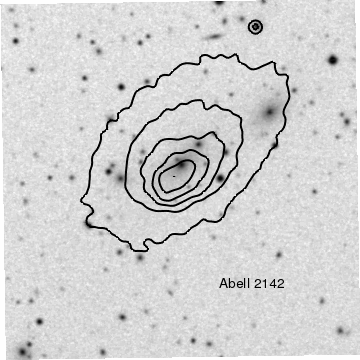Version: Postscript; PDF
```# Convert the pixel data from linear to log scaling
ivals = copy_piximgvals(dss)
set_piximgvals(dss, np.log10(ivals))

# Display the image, with black indicating high and white
# low pixel values

# Overlay the contours

# Add a label to the bottom-right of the plot

# Hide the axes and borders, make the plot fill the frame
hide_axis()
set_plot(["style","open"])
reposition_plot(0,0,1,1)
```

The read_file routine is used to read in the data from the file "a2142_dss.fits" into the dss object. We then replace the pixel values in dss by the logarithm of their values using the copy_piximgvals and set_piximgvals routines. The dss variable is then displayed with the add_image routine, and we set the invert_colormap attribute to True so that high values are displayed as black rather than white.

The above can also be achieved using the scale_image_crate routine from the CIAO contributed scripts package, for example:

```scale_image_crate(dss, "log10")
```

but the advantage of the manual approach is that the scaling is not limited to that provided by the scale_image_crate routine.

We could have used the following commands to display a logarithmically-scaled version of the optical data, but this would have lost the WCS information in the file header, which means we would not have been able to overlay the Chandra contours.

```dss = read_file("a2142_dss.fits")
ivals = copy_pixvals(dss)
```

The add_contour call overlays the contours onto the image; we chose to increase the width of the contours and to make them black so that they are visible both on the screen and in any hardcopy outputs created by print_window.

A label is positioned in the bottom-right corner of the plot, using the plot-normalized coordinate system; since the halign attribute is set to 0.5 the label is centered horizontally at x=0.7.

In order to remove the axes we have to call hide_axis, to hide both the X and Y axies, and set_plot to set the plot style to open, which hides the plot borders. Finally we call reposition_plot to make the plot fill the frame; an alternative method to achieve this - as used in the histogram overlay example - is to set the plot margins to 0.

## 2) The alpha channel can be used to blend together multiple images

In this example we show how overlapping images can be overlain, taking advantage of the alpha-channel support to make the top image partially transparent. Here we merge the X-ray observation of Abell 2142 with the digitized-sky survey image of the cluster.

The example also shows how you can adjust the threshold attribute of images to restrict the pixel range of the image being displayed.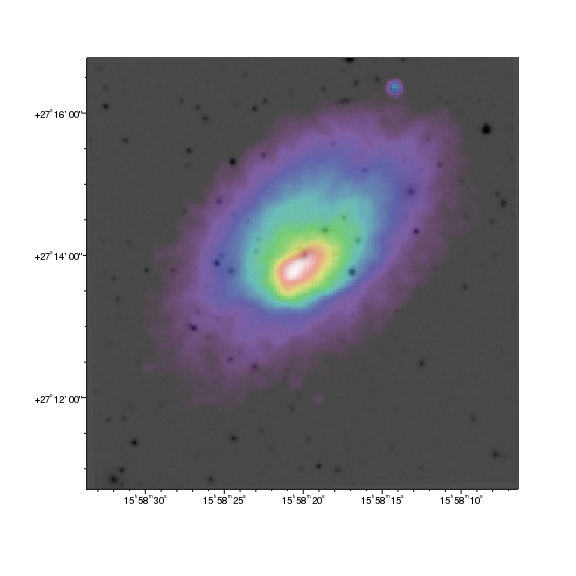Version: Postscript; PDF
```# Load in the background image
set_image(["invert_colormap",True])

# Ensure the axis is visible
axis = ChipsAxis()
axis.depth = 150
axis.majortick.style = "outside"
axis.minortick.style = "outside"
set_axis(axis)
set_xaxis(["tickformat","ra"])
set_yaxis(["tickformat","dec"])

# Overlay the X-ray data, restrict the range, and make it partly opaque
set_image(["threshold",[6, 60],"alpha",[0.7, 0.7]])
```

The second add_image call results in a plot which just shows the X-ray data, since - by default - images are fully opaque and so the original image (from the DSS) is obscured. The set_image call is used to change two image attributes that will "merge" the two images:

1. The alpha attribute takes a two-element array that describes how the alpha channel should vary with the image pixel values. Here we use [0.7, 0.7] to say that each pixel being displayed should have an alpha-channel value of 0.7.

If we had said [0.3, 1] then the opacity would have varied from 0.3 for pixels with the minimum displayed value to 1 for those with the maximum displayed value.

2. The threshold attribute is used to determine what range of pixel values is displayed by the colormap. Here, pixel values of 6, and below, are assigned to the minimum color whilst those with 60, or above, are given the maximum color. This range was selected to highlight the cluster emission whilst ignoring the X-ray background.

#### WCS projection support

In CIAO 4.10, WCS support in ChIPS is only available for tangent-plane projections (e.g. images with RA--TAN and DEC-TAN images). Other projections - such as the SIN projection - will be displayed in physical, or logical coordinates. For the gallery plots, SIN projection images (such as provided by NVSS) have been converted to TAN projection - using a combination of the remap program from WCSTools and the Montage package.

#### Opacity and Postscript output

Note that the postscript output created by print_window does not support opaque region or histogram fills; instead the opacity is taken to be 1. The relative depth of the objects can be changed - by altering the depth attribute or using the various "shuffle commands" (shuffle, shuffle_back, shuffle_front, shuffle_backward, shuffle_forward, and the set of shuffle_<object> routines) so that overlapping objects are not completely obscured if desired.

## 3) Show the different colormaps available within ChIPS

In this example we show the in-built colormaps that are available for displaying image data in ChIPS. The following example shows how you can also use your own colormaps.

This example also provides an example of how you can display a NumPy array as an image and write simple helper routines in Python to automate repetitive tasks.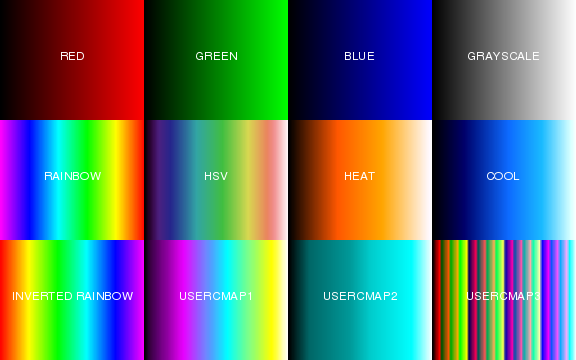Version: Postscript; PDF
```# Create a grid of plots to use, making sure they fill the frame
pref = ChipsPreferences()
pref.plot.leftmargin = 0
pref.plot.rightmargin = 0
pref.plot.topmargin = 0
pref.plot.bottommargin = 0
set_preferences(pref)
split(3,4)
current_plot("all")
set_plot(["style","open"])

# Add a simple image to each plot (a 1D array is shown
# as a 2D image where the Y axis has one element/pixel)
img = np.arange(1, 4097)
set_data_aspect_ratio("")
limits(Y_AXIS,0.5,1.5)
hide_axis()

pref = ChipsPreferences()
pref.label.color = "white"
pref.label.size = 16
pref.label.halign = 0.5
set_preferences(pref)

def doit(plot, cmap, invert=False, label=None):
"""Change the colormap of the plot to cmap and add a label
to the center of the plot. If label is None then the label is
set to the cmap value (converted to upper case). The invert
argument controls the 'invert_colormap' setting for the image.
"""
current_plot(plot)
set_image(["colormap", cmap, "invert_colormap", invert])
if label is None:
label = cmap.upper()

# Now change each plot
doit("plot1", "red")
doit("plot2", "green")
doit("plot3", "blue")
doit("plot4", "grayscale")
doit("plot5", "rainbow")
doit("plot6", "hsv")
doit("plot7", "heat")
doit("plot8", "cool")

# show the inverted version of the rainbow colormap
# directly beneath the original one (in plot5)
doit("plot9", "rainbow", invert=True, label="INVERTED RAINBOW")

# Now the user colormaps
doit("plot10", "usercmap1")
doit("plot11", "usercmap2")
doit("plot12", "usercmap3")
```

For this example we want to display a grid of plots - created by the split command - that fill the frame. Since the split command uses the default plot margins for determining how much of the frame to use when creating the grid, we first set the plot margins to 0 using the set_preferences routine. The add_window call is used to create a rectangular window; note that the on-screen size is not guaranteed to exactly match the requested size but that any postscript and PDF output will use this size (unless the fittopage attribute is set when calling print_window).

The set_plot call ensures that the following commands are applied to all the plots; this means, for instance, that the add_image call will create an image in each plot created by the split call.

Since we wish to display the different colormaps, we chose to display a simple image: a horizontal bar going from 1 to 4096. The plot is created with the data-aspect ratio set to 1:1. In this case this is not desired, so we use the set_data_aspect_ratio call to remove the setting, and change the Y-axis limits to cover just the image. An alternative way to set the Y-axis range would have use the image itself to set the range by saying:

```limits(Y_AXIS, chips_image, "imag1")
```

The label preferences are set to make sure any new label is drawn in white, with a font size of 16, and horizontally centered on the given location. These are set as preferences to avoid having to set them in each add_label call. We write a routine to automate setting the colormap and adding a label to each plot, imaginatively called doit. We take advantage of Python's "named keywords" when writing the function to support optional values; the routine defaults to using the upper-case version of the colormap name as the label text and not to invert the colormap, but these can be explicitly set with the label and invert arguments, as is done for the inverted-rainbow plot in plot9.

Each plot is selected, one of the default colormaps is chosen, and a label added - using the doit routine - to indicate which one is being used. The plots are arranged from left-to-right, and then top-to-bottom, by the split call; so plot1 is the plot in the top-left corner and plot4 is the plot in the top-right corner.

For the bottom-left plot (plot9), we use the same colormap as the plot above it - the rainbow map in plot5 - but invert the colormap.

The last three plots use the "user" colormaps - usercmap1, usercmap2, and usercmap3 - which default to the values shown here. The following example shows how these particular colormaps can be changed.

## 4) Using extra colormaps, read from a file

In this example we show how you can change the three "user" colormaps, using tables read in from disk files. For this we take advantage of the colormaps provided as part of CIAO for the dmimg2jpg tool, and accessible via the lut parameter file.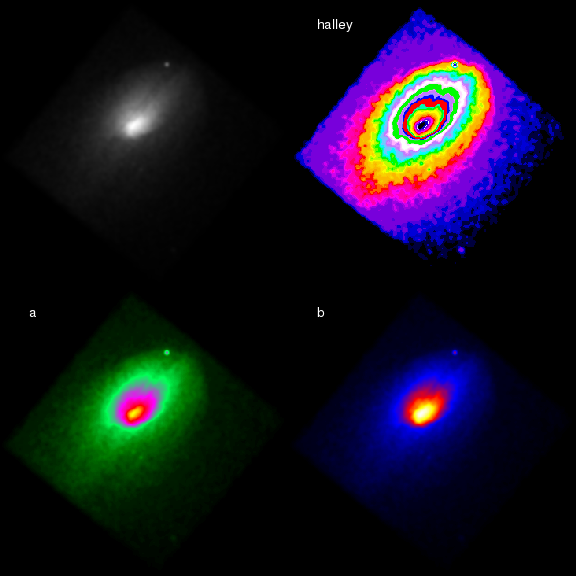Version: Postscript; PDF
```# Read in the halley, a, and b colormaps from CIAO
from paramio import *
halley = pget("lut", "halley")
a = pget("lut", "a")
b = pget("lut", "b")

# Create a grid of plots to use, making sure they fill the frame
pref = ChipsPreferences()
pref.plot.leftmargin = 0
pref.plot.rightmargin = 0
pref.plot.topmargin = 0
pref.plot.bottommargin = 0
set_preferences(pref)
split(2,2)
current_plot("all")
set_plot(["style","open"])

# Add the same image to each plot
hide_axis()

# Set the colormaps
current_plot("plot2")
set_image(["colormap","usercmap1"])

current_plot("plot3")
set_image(["colormap","usercmap2"])

current_plot("plot4")
set_image(["colormap","usercmap3"])

# Ensure all labels are visible in the hardcopy output
current_plot("all")
set_label(["color","white","size",18])
```

Here we decide to use the halley, a, and b colormaps from the lut parameter file. In order to get the file names, we load in the paramio module, and then use pget to query for the values. You can use the plist tool to view all the options (the actual location of the files depends on the location of your CIAO installation, here assumed to be /soft/ciao-4.7/):

```chips> !plist lut

Parameters for /soft/ciao-4.7/param/lut.par

(a = \${ASCDS_CALIB}/a.lut -> /soft/ciao-4.7/data/a.lut) Color lookup table
(aips = \${ASCDS_CALIB}/aips.lut -> /soft/ciao-4.7/data/aips.lut) Color lookup table
...
```

Once the file names have been retrieved, we use the load_colormap call to load in the data into the usercmap1, usercmap2, and usercmap3 colormap slots. If the location of the files are already known, or you want to use other files, you can call load_colormap directly: e.g.

```chips> load_colormap("fire.cmap")
```

where the format of the input file is described in the load_colormap help page.

Unlike the previous example, we use the Chandra observation of Abell 2142 as the image, and display it in a two by two grid of plots created by split. Rather than change the label preferences, we create the labels and then use the set_plot command to make sure all plots are current so that we can change the attributes of all the labels with a single call to set_label.

## 5) Creating a color map directly

Colormaps can also be created from NumPy or Python arrays as well as read from files. The chips_contrib.helix module, from the CIAO contributed scripts package, provides the load_colormap_cubehelix command, which takes advantage of this functionality to make it easy to use he CubeHelix scheme of Green, D. A., 2011, `A colour scheme for the display of astronomical intensity images', Bulletin of the Astronomical Society of India, 39, 289.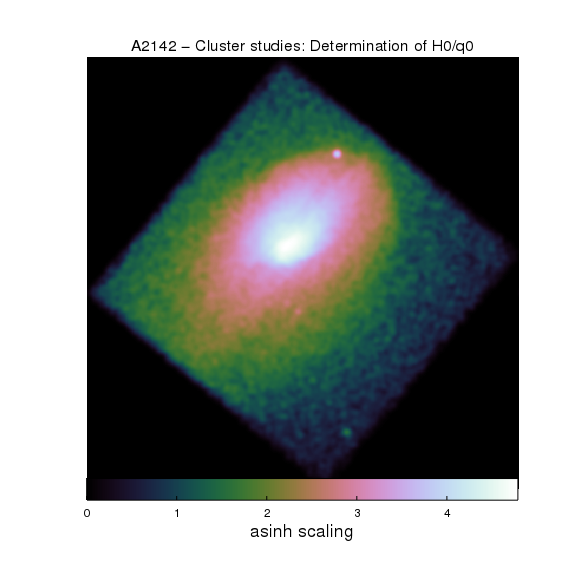Version: Postscript; PDF
```from crates_contrib.utils import scale_image_crate

# Convert to an 'asinh' scale for the image
scale_image_crate(cr, 'asinh')

# Display the image

# Create the plot title from the header keywords
title = cr.get_key_value('OBJECT') + ' - ' + cr.get_key_value('TITLE')
set_plot_title(title)
set_plot(["title.size",20])

# Hide the axes
hide_axis()
set_plot(["style","open"])

cbar = ChipsColorBar()
cbar.label.text = "asinh scaling"
cbar.label.size = 18
cbar.label.location = "outside"
cbar.offset.perpendicular = 20
set_colorbar(cbar)
```

The load_colormap_cubehelix() routine sets the chips_usercmap1 slot to contain the default CubeHelix scheme. We then apply this to the smoothed Abell 2142 data; unlike previous examples we use an 'asinh' scaling, provided by the scale_image_crate routine.

The remaining parts of the script set up the title, using the contents of the OBJECT and TITLE keywords in the header of the image, and a colorbar below the plot, taking care to hide the axes first.

## 6) Adjusting the alpha values to hide low-signal data

The load_colormap_cubehelix command used in the previous example combines the creation of the color map and its use in the load_colormap call. It is possible to do this manually, as shown below, where the CubeHelix colormap is adjusted so that the low end varies from being invisible (opacity=0) to fully opaque (opacity=1). The remaining elements, at higher signal levels, are all fully opaque. The idea here is to hide the low-level signal, allowing the background to show (in this case it is just the frame, so does not show anything but it avoids a large black region being displayed).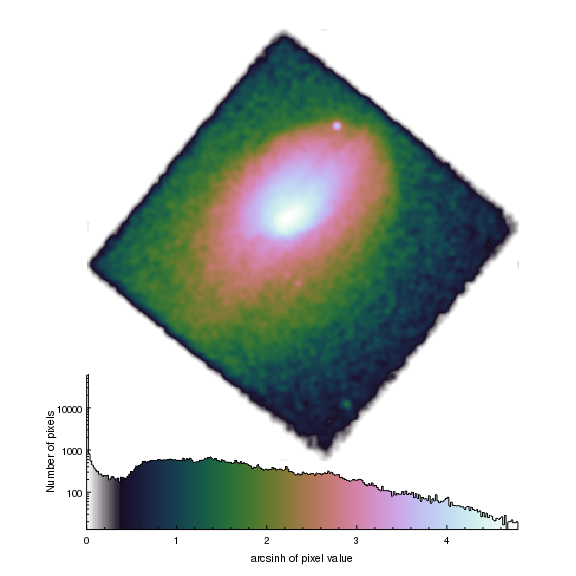Version: Postscript; PDF
```from crates_contrib.utils import scale_image_crate
from chips_contrib.helix import get_cubehelix

# Change the display preferences so screen and hardcopy
# outputs match.
set_preferences(["foreground.display","black","background.display","white"])

# Create the new colormap
(rs, gs, bs) = get_cubehelix()

# Now adjust the first 20 elements (~10%) to vary in opacity
# from 0 to 1 linearly, the remaining being fully opaque.
als = np.ones(rs.shape)
als[0:20] = np.arange(20) / 20.0

# Convert to an 'asinh' scale for the image
scale_image_crate(cr, 'asinh')

# Display the image
hide_axis()
set_plot(["style","open"])
move_plot(0,0.05,1)

# Add a histogram of the pixel distribution. Since ChIPS does not
# support a variable color map, create one histogram per bin
# (CubeHelix uses 256 bins by default).
#

(ys, edges) = np.histogram(cr.get_image().values, bins=256)
xlos = edges[:-1]
xhis = edges[1:]
irs = np.floor(rs * 255).astype(np.int16)
igs = np.floor(gs * 255).astype(np.int16)
ibs = np.floor(bs * 255).astype(np.int16)
colnames = ["{:02x}{:02x}{:02x}".format(ir,ig,ib) for (ir,ig,ib) in zip(irs,igs,ibs)]
set_preferences(["histogram.fill.style","solid","histogram.line.style","noline"])
for (xlo,xhi,y,colname,al) in zip(xlos,xhis,ys,colnames,als):
add_histogram([xlo], [xhi], [y], ['fill.color', colname, 'fill.opacity', al])

# Add a single histogram to outline the whole distribution
add_histogram(xlos, xhis, ys, ['fill.style', 'nofill', 'line.style', 'solid', 'id', 'outline'])

log_scale(Y_AXIS)
set_plot_xlabel("arcsinh of pixel value")
set_plot_ylabel("Number of pixels")
```

The get_cubehelix() routine is used to get the red, green, and blue components of the color scheme. An alpha channel - the variable a - is created to be the same size as these arrays, and with all values set to 1 (fully opaque). The first 20 elements are then changed so that the alpha channel ramps up linearly from 0 (first element) to 1 (twenty-first element) and then remains at 1 for the remaining elements. The choice over what range to do this scaling depends on the data; here 20 elements corresponds to almost 10 percent of the range and does not remove any of the important information.

The preferences for foreground.display and background.display are changed to make the opacity changes obvious on the screen display.

Note that most of the example code sets up the histogram display. Since ChIPS does not support multiple colors for curves or histograms, we create a histogram for each bin, setting its color by converting the 0-1 range of the red, green, and blue components into a hex-encoded string.

#### Opacity and Postscript output

Note that the postscript output created by print_window does not support opaque region or histogram fills; instead the opacity is taken to be 1. The relative depth of the objects can be changed - by altering the depth attribute or using the various "shuffle commands" (shuffle, shuffle_back, shuffle_front, shuffle_backward, shuffle_forward, and the set of shuffle_<object> routines) so that overlapping objects are not completely obscured if desired.

## 7) Overlay a plot on an image

In this example we display the Chandra image of Abell 2142 using the "physical" coordinate system, so that annotations (in this case lines) can be added to represent the regions used in the extraction of the radial profiles of the cluster. These radial profiles are then overlain on the image by adding a second plot and adding histograms to it.Version: Postscript; PDF
```add_image("a2142_smoothed.fits",["wcs","physical"])
plt = ChipsPlot()
plt.style = "open"
plt.leftmargin = 0
plt.rightmargin = 0
plt.topmargin = 0
plt.bottommargin = 0
set_plot(plt)
hide_axis()

# Indicate the boundary for the histograms

# Create a 200 pixel radius circle
theta = np.linspace(0, 2*np.pi, 60)
xr = 3786 + 200 * np.cos(theta)
yr = 3767 + 200 * np.sin(theta)

# Create a new plot for the histograms

log_scale()
limits(X_AXIS,10,400)

# Indicate the radius of the circle in the image

# Ensure the axes are visible in the hardcopy output
set_axis(["*.color","white"])
```

The wcs attribute is set to physical in the add_image call to avoid using the world coordinate system for the axes: e.g. as used in the first image example. The set_plot and hide_axis calls are used to make sure the image fills the frame, and to hide both the axes and plot borders (see the earlier overlay-a-contour example for an alternative method for this, using the reposition_plot routine).

The image is then annotated with a vertical dashed line and labels, used to indicate the regions used in creating the radial profiles, as shown by the use of dmhistory below:

```unix% dmhistory rprof.n.fits dmextract action=pset
unix% pget dmextract infile
evt2.fits[sky=rect(0,3767,8000,8000)][bin sky=annulus(3786,3767,0:380:4)]
```

and

```unix% dmhistory rprof.s.fits dmextract action=pset
unix% pget dmextract infile
evt2.fits[sky=rect(0,0,8000,3767)][bin sky=annulus(3786,3767,0:380:4)]
```

In order to provide some idea of the image scale, a circle of radius 200 pixels is drawn - in orange - about the center of the radial profiles. In CIAO 4.7 there is no native support for drawing a circle, so we approximate a circle as a set of line segments evaluated at 60 points around the circle. An alternative approach would have been to use the add_region command, and its support for regular-sided polyhedra to approximate a circle: for example

```chips> add_region(60, 3786, 3767, 200/np.sqrt(2), ["fill.style", "none", "line.color", "gold"])
```

where 60 gives a similar number of vertices to the line version, and the sqrt(2) term is needed to get a circular radius of 200 pixels. The advantage of this approach is that the region is automatically closed, whereas you have to manually ensure it is closed using the line version.

We now add a plot to the bottom-half of the plot, and set its style to open so that the plot borders are not displayed (this removes the top and right borders from the display). The two radial profiles are added as red and green histograms, and the axis limits and scaling changed. A vertical line is added at a radius of 200 pixels in orange to match the circle drawn on the image.

Finally we use the *.color attribute to change the color attribute of all elements of both axes to white so that they will be visible in the hardcopy output created by print_window.

## 8) WCS Axis grids

This example shows the support for drawing axis grid lines for axes that represent a WCS projection. The image is the 0.5 to 7 keV data for ObsId 3477, which was taken as a follow-up of the 020321 Gamma-Ray Burst. As an example of including annotations in such plot we mark on the location of one of the initial estimates of the burst position.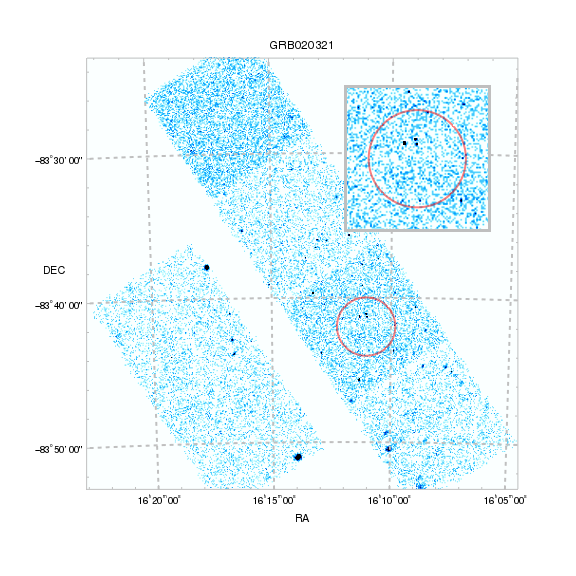Version: Postscript; PDF
```# Create the window and display the image in it
make_figure("img.3477.fits","image",["depth",50])
img = ChipsImage()
img.colormap = "cool"
img.threshold = [0, 5]
img.invert_colormap = True
img.interpolation = "bilinear"
set_image(img)

# Add in the major-grid lines
set_axis(["majorgrid.visible",True,"majorgrid.thickness",2])
set_xaxis(["tickformat","ra"])

# In CIAO 4.6 you have to say y.label.angle rather than label.angle
set_yaxis(["tickformat","dec","y.label.angle",0])

set_axis("all",["*.color","gray"])
set_axis(["ticklabel.color","default","label.color","default"])

# Mark on the location from http://gcn.gsfc.nasa.gov/gcn3/1285.gcn3
x0 = 242.76
y0 = -83.70
r = 2.0 / 60 / np.cos(y0*np.pi/180)

# Create an inset plot of this region
set_image(img)

# zoom into the region of interest
panto(x0,y0)
zoom(5)

# Hide the axis information and make sure the border is visible
hide_axis()
set_plot(["style","boxed"])
set_axis("all",["depth",110,"*.color","gray","thickness",3])
```

As with the COSMOS survey example, we create a window where the default colors for the on-screen display are set to match the hardcopy versions. This can be useful to check that the chosen color schemes will still be visible in the output created by print_window.

Once the window has been created the make_figure and set_image commands are used to set up the image. We use an Attribute Object - the img object - to set the image properties since we will re-use it later for the inset plot. Note that the image was created from the archival "event 2" file using the command:

```unix% dmcopy "acisf03477N002_evt2.fits[energy=500:7000][bin x=1932.5:5565.5:8,y=2214.5:6321.5:8]" \
img.3477.fits
```

The image is set to a depth of 50 so that it does not overlap the axis elements, in particular the majorgrid lines that are turned on by the

`set_axis(["majorgrid.visible", True])`

line. Changing the format used for the ticklabels - namely setting tickformat to ra and dec - also changes the location of these gridlines. All axis elements are set to gray, using the *.color short form, and then the labels, which lie outside the plot area, are set back to the default color (which happens to be black).

The add_region command is used to draw a circle indicating the two-arcminute uncertainty in the location from the GCN circular; we are actually approximating a circle by a 101-sided regular polyhedron.

To provide a close up of this region we add a second plot, within the first one, using the add_plot command, specifying the desired plot location in frame-normalized coordinates. Some trial and error may be required to get sensible values; you can either use undo and change the coordinates in the add_plot call or use either move_plot or reposition_plot to change the plot location.

Once the plot has been added, we add the same image to it as in the first plot, this time using the add_image command since we are not interested in the extra annotations that make_figure provides. Since the image fills the full area of the second plot we do not need to worry about contents of the first plot appearing through the plot as we did in the COSMOS survey example, which is why we did not need to create a second frame here.

Once the same error circle has been drawn we use the panto and zoom commands to change the image area displayed by the plot (for this example a zoom of 500% is a good match to the 4 arcminute diameter of the error circle).

Once the image area has been changed we finish by tidying up the second plot; the axes are hidden and the plot style changed to boxed, then the border axes have their thickness and color changed to provide visual distinction between the two plots.

## 9) Create a grid of thumbnail images of Chandra sources

Here we display a set of 8 thumbnails of Chandra sources from an observation in a three-by-three grid. Each plot is annotated with a label and scale bar, and the position of sources detected by 2MASS are marked.

It also shows how you can use the open_undo_buffer() and close_undo_buffer() routines from the ChIPS advanced module to make a set of commands act as a single command (for undo and redo purposes), by taking advantage of the chips_contrib.decorators.add_chips_undo_buffer routine.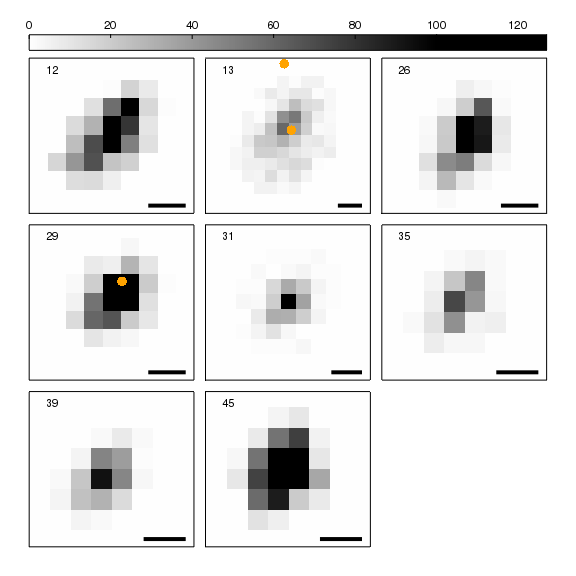Version: Postscript; PDF
```

"Display an image of the source, with label and scale bar"

fname = "img.315.fits[sky=region(src.315.fits[component={}])]".format(src)
iopts = { "colormap": colormap, "invert_colormap": invert,
"depth": 50, "threshold": [0, maxval]}

add_label(0.1, 0.9, str(src), {"coordsys": PLOT_NORM, "size": 14})

# Find the bottom-right corner of the image in data coordinates
[x, y] = convert_coordinate([0.95, 0.05], PLOT_NORM, DATA)

# How many degrees to represent 1 arcsecond?
dx = 1.0 / (3600 * np.cos(y * np.pi / 180))

add_line(x+dx, y, x, y, {"thickness": 4})

# Use the Python decorator to add the undo/redo buffer handling to
# this routine.
def thumbnails(srcs, maxval, invert=True, colormap="grayscale"):
"""Create a square set of plots to display the thumbnail images for the
source list given by srcs.

All images are thresholded to display the pixel range 0 to maxval.

The image and source list are hard-coded to img.315.fits and src.315.fits.
"""

nsrc = len(srcs)
ngrid = np.ceil(np.sqrt(nsrc)).astype(np.int)

# Set up the borders so that the grid_objects call fills the frame
set_preferences(["plot.leftmargin", "0.05",
"plot.rightmargin", "0.05",
"plot.bottommargin", "0.05",
"plot.style", "boxed",
"foreground.display", "black",
"background.display", "white"])

# Create the thumbnail images and then arrange them into a grid
pnames = []
for src in srcs:
pname = "src{}".format(src)
pnames.append(pname)

grid_objects(ngrid, ngrid, 0.02, 0.02, 0, pnames)

# hide all the axes
current_plot("all")
hide_axis()

# As we want the colorbar to span all columns of the grid
# we need to calculate the length, which is in plot-normalized
# coordinates for the first plot.

# We start by calculating the width of the bar in frame-normalized
# coordinates:
current_plot(pnames[ngrid-1])
rframe2 = convert_coordinate(1, PLOT_NORM, FRAME_NORM, X_AXIS)
current_plot(pnames)
lframe1 = convert_coordinate(0, PLOT_NORM, FRAME_NORM, X_AXIS)

# Now we need to width of the current plot in frame-normalized
# coordinates so that we can convert to plot-normalized
# coordinates for the color-bar length.
rframe1 = convert_coordinate(1, PLOT_NORM, FRAME_NORM, X_AXIS)

clen = (rframe2 - lframe1) / (rframe1 - lframe1)
add_colorbar(0, 1.1, {"halign": 0, "length": clen, "width": 0.1})

# Call the routine
thumbnails([12, 13, 26, 29, 31, 35, 39, 45], 100)

current_plot("all")
set_curve(["line.style", "noline", "symbol.style", "circle", "symbol.color", "orange"])
```

We have split the task up into two basic parts, an add_thumbnail routine to add the image and the thumbnails() driver routine. With both these routines set up, the visualization is created with a call to thumbnails and then a few calls to set up the 2MASS sources.

We start with the add_thumbnail routine which - given a source number and maximum pixel value - displays an image of the source, and adds both a label and a line (representing 1 arcsecond). The label is positioned in the top-left corner of the plot, using the plot-normalized coordinate system. We want the scale bar - which has a length of one arcsecond - to end at the bottom-right corner of the plot, five percent in from both edges; in other words at a plot-normalized coordinate of (0.95,0.05). Converting these to the data system used by the plot allows us to calculate the length of the bar, correcting for the declination of the source.

The second part - represented by the thumbnails() routine - is the driver routine. Given a set of sources, and a maximum pixel value, it sets up the plots, adds the thumbnails, arranges the grid of plots, and sets up the color bar across the top.

This routine takes advantage of Python decorators to use the add_chips_undo_buffer routine from the chips_contrib.decorators module - provided as part of the CIAO contributed-script package - so that calling this routine will act as if it is a single ChIPS command. That is:

1. will only display the final visualization to the user, so that you do not see all the intermediate steps (it acts as if the window.display setting is False until the close_undo_buffer call),

2. if the commands were successful, they will be treated as a single ChIPS command by the undo and redo routines,

3. and if there was a problem then all the ChIPS commands made up to that point (by the example) will be forgotten, so that the previous visualization will be unchanged.

The set_preferences call is used to change the plot preferences so that a single plot fills most of the frame, which will be used by the grid_objects call later on, and to make the on-screen display use the same colors as the hardcopy versions for the default color and bgcolor values.

For each source we create a plot - with the name src#, where # is the source number - and add the thumbnail to it. At this point the plots all overlap, so we use the grid_objects routine to create a square grid of plots. We could instead have used the split command before the add_thumbnail calls - but then would have had to delete any excess plots (i.e. for those cases when the number of sources is not a square number).

After re-arranging the plots we hide the axes in all the plots, leaving just an outline. As the images were created with a depth less than 100 these plot edges will be visible.

The grid is finished by adding a colorbar above the plots. Since the colorbar is associated with an image, we add it to the first plot, using halign=0 together with an x position of 0 to start the bar at the left edge of the plot. The length of the bar - here given in the cbar variable - is calculated so that the bar extends across all the columns in the grid of plots. The length is defined in the plot-normalized coordinate system of the first plot, so we use the frame-normalized coordinate system, together with convert_coordinate, to convert between the different coordinate systems in use.

After all the X-ray images have been created we mark on sources from the 2MASS catalogue. The file 2mass.315.tsv was created as a tab-separated ASCII file using the catalog support in ds9, and contains the source locations in the RAJ2000 and DEJ2000 columns. Since we have displayed the images using the EQPOS coordinate system, we can just add the catalog values as a curve (the [opt kernel=text/tsv] fragment is needed since this format can not be guessed by the support for ASCII files in the CIAO Data Model). By setting the plot currency to "all" before the add_curve call we add the points to all plots (even though in this case only two plots actually contain a matching source).

The output of the info command after this visualiation looks like:

```Window [win1]
Frame [frm1]
Plot [src12]   (0.05,0.63)  .. (0.34,0.90)
Border bottom [bx1]  top [bx2]  left [by1]  right [by2]
Image [image1]
X Axis [ax1]
Y Axis [ay1]
Label [lbl1]
Line [line1]
ColorBar [cbar1]
Curve [2mass]
Plot [src13]   (0.36,0.63)  .. (0.64,0.90)
Border bottom [bx1]  top [bx2]  left [by1]  right [by2]
Image [image1]
X Axis [ax1]
Y Axis [ay1]
Label [lbl1]
Line [line1]
Curve [2mass]
Plot [src26]   (0.66,0.63)  .. (0.95,0.90)
Border bottom [bx1]  top [bx2]  left [by1]  right [by2]
Image [image1]
X Axis [ax1]
Y Axis [ay1]
Label [lbl1]
Line [line1]
Curve [2mass]
Plot [src29]   (0.05,0.34)  .. (0.34,0.61)
Border bottom [bx1]  top [bx2]  left [by1]  right [by2]
Image [image1]
X Axis [ax1]
Y Axis [ay1]
Label [lbl1]
Line [line1]
Curve [2mass]
Plot [src31]   (0.36,0.34)  .. (0.64,0.61)
Border bottom [bx1]  top [bx2]  left [by1]  right [by2]
Image [image1]
X Axis [ax1]
Y Axis [ay1]
Label [lbl1]
Line [line1]
Curve [2mass]
Plot [src35]   (0.66,0.34)  .. (0.95,0.61)
Border bottom [bx1]  top [bx2]  left [by1]  right [by2]
Image [image1]
X Axis [ax1]
Y Axis [ay1]
Label [lbl1]
Line [line1]
Curve [2mass]
Plot [src39]   (0.05,0.05)  .. (0.34,0.32)
Border bottom [bx1]  top [bx2]  left [by1]  right [by2]
Image [image1]
X Axis [ax1]
Y Axis [ay1]
Label [lbl1]
Line [line1]
Curve [2mass]
Plot [src45]   (0.36,0.05)  .. (0.64,0.32)
Border bottom [bx1]  top [bx2]  left [by1]  right [by2]
Image [image1]
X Axis [ax1]
Y Axis [ay1]
Label [lbl1]
Line [line1]
Curve [2mass]
```

#### Using the ChIPS buffer routines directly

The add_chips_undo_buffer() routine can be removed and replaced with:

1. The initial import of the "advanced" ChIPS module:

`import pychips.advanced as adv`
2. Placing the ChIPS commands in the thumbnails routine within the following try/except/else block:

```adv.open_undo_buffer()
try:
... ChIPS commands ...
except: